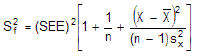### CFA Practice Question

There are 985 practice questions for this topic.

### CFA Practice Question

Which of the following is not a factor in reducing the variance of the predicted errors for the dependent variable given an estimated regression model?

A. An increase in the value of the regression coefficient.
B. A decrease in the standard error of estimate (SEE).
C. The value of the predicted independent value being closer to its mean.